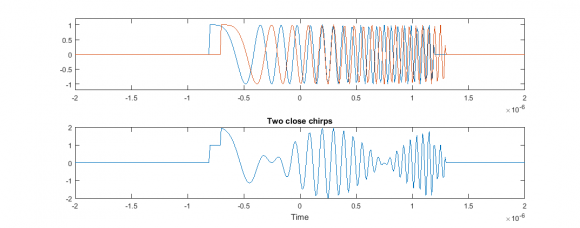## Verification of range resolution using simulationsStarted by 7 years ago3 replieslatest reply 7 years ago240 views

Hi all

I am working on Match Filter/Correlation algorithms for pulsed radar/sonar. The literature says that if we use CW Pulse, the range resolution is defined by pulse length 'T' according to formula (cT/2, where c=sound speed) and if we use broadband pulses like linear frequency modulated or hyperbolic frequency modulated (LFM or HFM), we get range resolution according to formula (c/2B) where B is signal Bandwidth.

Now i am trying to do some MATLAB simulations to verify these range resolutions. I have generated LFM/HFM pulses using Matlab 'chirp' function but i am confused how to use these pulses in the way so that i can get MF output with desired resolution i.e. c/2B*.

Kindly help me how to generate simulated data with desired effects as an input for MF algorithm

*Note: According to my understanding, if two objects (from which LFM/HFM pulse is reflected) are placed c/2B m apart, i should get two distinguishable peaks at MF output.

[ - ]Hi,

Is the question about how to produce two delayed chirps or how to matched filter them or how to decide if the second chirp can be resolved?

At small delays the matched filter outputs for both reflections start to interfere and you can not reliably resolve them if they are closer than about 1.4 c/2B.

[ - ]Dear Krasin

Thanks for reply. My question was how to produce simulated input (having c/2B range separation b/w them) to match filter.

[ - ]Hi Naumankalia,

To produce a delayed chirp (with whatever delay you want) you need to:

1. Delay the chirp, e g. instead of  chirp(t, f0, T, f1)   you need  chirp(t - t1, f0, T, f1) .
2. Delay the envelope of the chirp, i.e. the period over which it is calculated. Matlab will produce a chirp over the whole time t that you put in the chirp() function but you need only a chirp with duration T.
3. Multiply the chirp and the envelope window.

To achieve output with range separation t2 - t1, repeat the procedure with a chirp with delay t2 and sum both chirps.

Example implementation:

%% Example parameters
fs = 100e6; % sampling frequency
T = 2e-6; % chirp duration
B = 20e6; % chirp bandwidth
f0 = 10e6; % frequency at time zero
f1 = f0 + B/2;
N = 200;
n = -N:N; % index
t = n/fs; % time axis

% zero centred chirp with duration T
y = chirp(t,f0,T/2,f1); % zero centred chirp
w0 = rectpuls(t, T); % zero centred window with span T
y0 = y.*w0;
plot(t, y, t, y0), ylim([-1.2, 1.2])

% delayed by t1
t1 = 2e-7;
y1 = chirp(t-t1, f0, T/2, f1); % delayed chirp
w1 = rectpuls(t-t1, T); % delayed window
y1 = y1.*w1; % finite duration chirp

% delayed by t2
t2 = 3e-7;
y2 = chirp(t-t2, f0, T/2, f1);
w2 = rectpuls(t-t2, T);
y2 = y2.*w2;

% both chirps
y_both = y1 + y2;
plot(t, y1, t, y2, t, y_both)Much simpler approach is to convolve the chirp with a signal of appropriately delayed impulses (this way you can introduce a delay of a whole number of sampling intervals):

target = zeros(1,M);
target(t1/fs) = 1;
target(t2/fs) = 1;
y_both = conv(target, y);


The number 1.4 is based on my simulations, but it corresponds to the Rayleigh criterion (https://en.oxforddictionaries.com/definition/rayle...), i.e. the position of the first minimum of the sinc function. If you need to go deeper, check the Rihaczek paper "Radar resolution of ideal point scatterers" http://ieeexplore.ieee.org/document/489527/ . He has very good books on high resolution radars also.